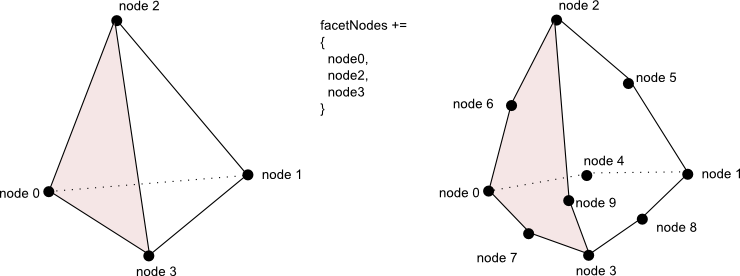## Interface MiVolumeCell

• ### Method Summary

All Methods
Modifier and Type Method Description
`double[]` ```getFacetCenter​(int facet, MiGeometryI geometry)```
Gets the center of the specified cell facet.
`int[]` ```getIsosurfTopology​(int caseId, int[] edges)```
Returns an array containing the topological polygons defining the part of the isosurface topology in this cell.
`int` ```getMarchingCaseId​(boolean[] nodesSign, long beginNodeId)```
Gets the isosurface "marching cube" entry according to the sign of each node in the cell.
`long[]` ```getNodesIndexOfFacet​(int facet, long[] facetNodes)```
Returns an array containing node indices belonging to a given facet.
`int` `getNumFacets()`
Gets the number of facets.
• ### Methods inherited from interface com.openinventor.meshvizxlm.mesh.cell.MiCell

`getCenter, getNodeIndex, getNumNodes, getRelativeSize, isPointInsideCell`
• ### Method Detail

• #### getNumFacets

`int getNumFacets()`
Gets the number of facets.
Returns:
number of facets of this cell
• #### getNodesIndexOfFacet

```long[] getNodesIndexOfFacet​(int facet,
long[] facetNodes)```
Returns an array containing node indices belonging to a given facet.

If the specified array length is equals to the number of node indices to be returned, they are returned therein. Otherwise, a new array is allocated.

For instance an implementation of this method for a linear tetrahedron cell like the following oneshould return an array filled by = {node0,node2,node3} if the facet argument corresponds to the pink one (Provided that `MiCell.getNodeIndex(int)` follows this tetrahedron node numbering.)

Parameters:
`facet` - The index of the facet (where `0 <= facet < getNumFacets`).
`facetNodes` - The array into which the node indices of the facet are to be stored if its length is equals to the number of node indices to be returned; otherwise, a new array is allocated for this purpose.
Returns:
an array containing the node indices of the facet.
• #### getIsosurfTopology

```int[] getIsosurfTopology​(int caseId,
int[] edges)```
Returns an array containing the topological polygons defining the part of the isosurface topology in this cell.

This method is used during isosurface and slice extraction on an unstructured mesh (for instance `MoMeshPlaneSlice`, `MoMeshIsosurface`, `MiIsosurfExtractUnstructured` ). Static implementations are provided for the most common basic volume cells:

If the specified array is large enough to contain the number of node indices to be returned, they are returned therein. Otherwise, a new array is allocated.

Parameters:
`caseId` - the isosurf "marching cube" entry for this cell. This is the value returned by getMarchingCaseId
`edges` - The array into which the node indices defining the isosurf topology in this cell are to be stored if its length is large enough; otherwise, a new array is allocated for this purpose. New pairs of node ids are stored into the array, each pair of nodes must correspond to an edge of the cell which is cut by the isosurface. An edge is cut by the isosurface when the two nodes of the edge have different signs. The pair of nodes must be correctly ordered to define a non manifold polygon. When the isosurface part is made up of several polygons, each polygon must be separated by `MxMeshViz.END_LIST`. The end of the polygons list must be indicated by a pair of `MxMeshViz.END_LIST`.
Returns:
an array containing the topological polygons defining the part of the isosurface topology in this cell. The function may return null only for polyhedral cells.
• #### getMarchingCaseId

```int getMarchingCaseId​(boolean[] nodesSign,
long beginNodeId)```
Gets the isosurface "marching cube" entry according to the sign of each node in the cell.

The return entry corresponds to the first argument of `getIsosurfTopology(int, int[])` This method is used during isosurface and slice extraction on an unstructured mesh (for instance `MoMeshPlaneSlice`, `MoMeshIsosurface`, `MiIsosurfExtractUnstructured` ).

Note: MeshViz XLM assumes that the cell does not contain any part of an isosurf if and only if the "marching cube" entry equals 0. Thus if all the bits in this word are 1, the method returns 0.

Parameters:
`nodesSign` - An array containing as many booleans as the number of nodes in the mesh. `nodesSign[i]` is relative to the sign of the isovalue-i-th node's value.
`beginNodeId` - is the first node id used by the mesh topology (see `MiTopologyExplicitI.getBeginNodeId()` .
Returns:
a binary word containing as many bits as the number of nodes in the cell; the i-th bit in the returned word equals `nodesSign[getNodeIndex(i)]`.
• #### getFacetCenter

```double[] getFacetCenter​(int facet,
MiGeometryI geometry)```
Gets the center of the specified cell facet.

This method is not used by all extraction classes.

Parameters:
`facet` - index of the facet (where `0 <= facet < getNumFacets`).
`geometry` - geometry of the mesh, it is used to retrieve the node coordinates of this cell
Returns:
the center of the specified cell facet.